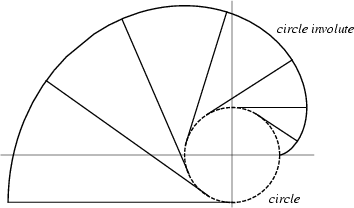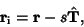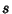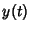## InvoluteAttach a string to a point on a curve. Extend the string so that it is tangent to the curve at the point of attachment. Then wind the string up, keeping it always taut. The Locus of points traced out by the end of the string is the involute of the original curve, and the original curve is called the Evolute of its involute. Although a curve has a unique Evolute, it has infinitely many involutes corresponding to different choices of initial point. An involute can also be thought of as any curve Orthogonal to all the Tangents to a given curve.

The equation of the involute is(1)

whereis the Tangent Vector(2)

andis the Arc Length(3)

This can be written for a parametrically represented functionas(4)(5)

 Curve Involute Astroid Astroid 1/2 as large Cardioid Cardioid 3 times as large Catenary Tractrix Circle Catacaustic for a point source Limaçon Circle Circle Involute (a Spiral) Cycloid equal Cycloid Deltoid Deltoid 1/3 as large Ellipse Ellipse Involute Epicycloid reduced Epicycloid Hypocycloid similar Hypocycloid Logarithmic Spiral equal Logarithmic Spiral Neile's Parabola Parabola Nephroid Cayley's Sextic Nephroid Nephroid 2 times as large

References

Cundy, H. and Rollett, A. Roulettes and Involutes.'' §2.6 in Mathematical Models, 3rd ed. Stradbroke, England: Tarquin Pub., pp. 46-55, 1989.

Dixon, R. String Drawings.'' Ch. 2 in Mathographics. New York: Dover, pp. 75-78, 1991.

Gray, A. Involutes.'' §5.4 in Modern Differential Geometry of Curves and Surfaces. Boca Raton, FL: CRC Press, pp. 81-85, 1993.

Lawrence, J. D. A Catalog of Special Plane Curves. New York: Dover, pp. 40-42 and 202, 1972.

Lee, X. Involute.'' http://www.best.com/~xah/SpecialPlaneCurves_dir/Involute_dir/involute.html.

Lockwood, E. H. Evolutes and Involutes.'' Ch. 21 in A Book of Curves. Cambridge, England: Cambridge University Press, pp. 166-171, 1967.

Pappas, T. The Involute.'' The Joy of Mathematics. San Carlos, CA: Wide World Publ./Tetra, p. 187, 1989.

Yates, R. C. Involutes.'' A Handbook on Curves and Their Properties. Ann Arbor, MI: J. W. Edwards, pp. 135-137, 1952.